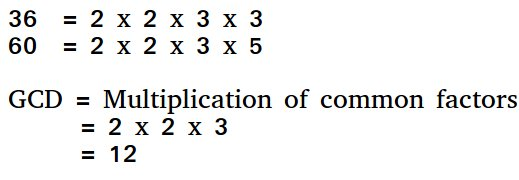# Program to find HCF iteratively

HCF (Highest Common Factor) or GCD (Greatest Common Divisor) of two numbers is the largest number that divides both of them.For example GCD of 20 and 28 is 4 and GCD of 98 and 56 is 14.

## Recommended: Please solve it on “PRACTICE ” first, before moving on to the solution.

We have discussed recursive solution in below post.Recursive program to find GCD or HCF of two numbers

Below is iterative implementation of Euclid’s algorithm

## C++

 `// C++ program to find HCF of two  ` `// numbers iteratively.  ` `#include ` `using` `namespace` `std; ` ` `  `int` `hcf(``int` `a, ``int` `b)  ` `{  ` `    ``while` `(a != b)  ` `    ``{  ` `        ``if` `(a > b)      ` `            ``a = a - b;      ` `        ``else`     `            ``b = b - a;      ` `    ``}  ` `    ``return` `a;  ` `}  ` ` `  `// Driver code ` `int` `main()  ` `{  ` `    ``int` `a = 60, b = 96;  ` `    ``cout << hcf(a, b) << endl;  ` `    ``return` `0;  ` `}  ` ` `  `// This code is contributed by shubhamsingh10 `

## C

 `// C program to find HCF of two ` `// numbers iteratively. ` `#include ` ` `  `int` `hcf(``int` `a, ``int` `b) ` `{ ` `    ``while` `(a != b) { ` `        ``if` `(a > b)         ` `            ``a = a - b;         ` `        ``else`         `            ``b = b - a;         ` `    ``} ` `    ``return` `a; ` `} ` `int` `main() ` `{ ` `    ``int` `a = 60, b = 96; ` `    ``printf``(``"%d\n"``, hcf(a, b));  ` `    ``return` `0; ` `} `

## Java

 `// JAVA Code for Program to find  ` `// HCF iteratively ` `import` `java.util.*; ` ` `  `class` `GFG { ` `     `  `    ``static` `int` `hcf(``int` `a, ``int` `b) ` `    ``{ ` `        ``while` `(a != b) ` `        ``{ ` `            ``if` `(a > b)         ` `                ``a = a - b;         ` `            ``else`        `                ``b = b - a;         ` `        ``} ` `        ``return` `a; ` `    ``} ` `     `  `    ``/* Driver program */` `    ``public` `static` `void` `main(String[] args)  ` `    ``{ ` `         ``int` `a=``60``, b=``96``; ` `         ``System.out.println(hcf(a, b));  ` ` `  `    ``} ` `} ` ` `  `// This code is contributed by Arnav Kr. Mandal. `

## Python3

 `/``/` `Python program to find HCF of two ` `/``/` `numbers iteratively. ` `def` `hcf(a, b): ` `    ``while` `(a !``=` `b): ` `        ``if` `(a > b): ` `            ``a ``=` `a ``-` `b ` `        ``else``: ` `            ``b ``=` `b ``-` `a ` `    ``return` `a ` `     `  `a ``=` `60` `b ``=` `96` `print``(hcf(a, b)) `

## C#

 `// C# Code for Program to find  ` `// HCF iteratively ` `using` `System; ` ` `  `class` `GFG { ` `     `  `    ``static` `int` `hcf(``int` `a, ``int` `b) ` `    ``{ ` `        ``while` `(a != b) ` `        ``{ ` `            ``if` `(a > b)  ` `                ``a = a - b;  ` `            ``else` `                ``b = b - a;  ` `        ``} ` `        ``return` `a; ` `    ``} ` `     `  `    ``// Driver program  ` `    ``public` `static` `void` `Main()  ` `    ``{ ` `        ``int` `a = 60, b = 96; ` `        ``Console.WriteLine(hcf(a, b));  ` ` `  `    ``} ` `} ` ` `  `// This code is contributed by vt_m. `

## PHP

 ` ``\$b``)      ` `            ``\$a` `= ``\$a` `- ``\$b``;      ` `        ``else` `            ``\$b` `= ``\$b` `- ``\$a``;      ` `    ``} ` `     `  `    ``return` `\$a``; ` `} ` ` `  `// Driver code ` `\$a` `= 60; ``\$b` `= 96; ` `echo` `hcf(``\$a``, ``\$b``), ``"\n"``;  ` `     `  `// This code is contributed by ajit ` `?> `

Output:

`12`

Attention reader! Don’t stop learning now. Get hold of all the important DSA concepts with the DSA Self Paced Course at a student-friendly price and become industry ready.

My Personal Notes arrow_drop_upCheck out this Author's contributed articles.

If you like GeeksforGeeks and would like to contribute, you can also write an article using contribute.geeksforgeeks.org or mail your article to contribute@geeksforgeeks.org. See your article appearing on the GeeksforGeeks main page and help other Geeks.

Please Improve this article if you find anything incorrect by clicking on the "Improve Article" button below.

Improved By : jit_t, SHUBHAMSINGH10

Article Tags :
Practice Tags :

Be the First to upvote.

Please write to us at contribute@geeksforgeeks.org to report any issue with the above content.